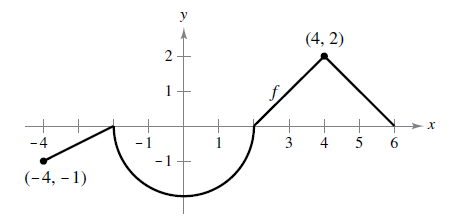Chapter 4.3, Problem 47E

Chapter
Section
Textbook Problem

Think About It The graph of f consists of line segments and a semicircle, as shown in the figure. Evaluate each definite integral by using geometric formulas.(a) ∫ 0 2 f ( x ) d x (b) ∫ 0 6 f ( x ) d x (c) ∫ − 4 2 f ( x ) d x (d) ∫ − 4 6 f ( x ) d x (e) ∫ − 4 6 | f ( x ) | d x (f) ∫ − 4 6 [ f ( x ) + 2 ] d x

(a)

To determine

To calculate: The integral 02f(x)dx using the graph.

Explanation

Given:

The graph of f consists of line segments and a semicircle

Formula used:

If f is continuous and non-negative on the closed interval [a,b] then the area of the region bounded by the graph of f, the x-axis and the vertical lines x=a,x=b that depict the border points. Then,

Area=abf(x)dx

Calculation:

The integral 02f(x)dx

(b)

To determine

To calculate: The integral 26f(x)dx using the graph.

(c)

To determine

To calculate: The integral 42f(x)dx using the graph.

(d)

To determine
The integral 46f(x)dx using the graph.

(e)

To determine

To calculate: The integral 46|f(x)|dx using the graph.

(f)

To determine

To calculate: The integral 46[f(x)+2]dx using the graph.

Still sussing out bartleby?

Check out a sample textbook solution.

See a sample solution

The Solution to Your Study Problems

Bartleby provides explanations to thousands of textbook problems written by our experts, many with advanced degrees!

Get Started

Find more solutions based on key concepts